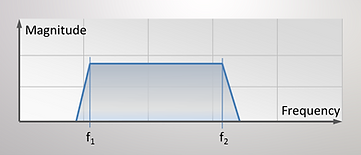What is Vibration?

# Basics of Vibration 3

## The Vibration Spectrum​

### ​​Frequency Analysis of Periodic Vibration

We have looked at sinusoidal vibration so far. Real vibration however normally consists of many different frequencies with different amplitudes occurring simultaneously. The vibration signal might  then look like on the picture below. It is not sinusoidal however it looks periodic!By applying the method of Fourier the signal above can be split up in its components and visualized by a so-called frequency analysis. The picture opposite illustrates this.

On the left side we find our periodic vibration signal and then the all Fourier components as sinusoidal signals with their frequency and magnitude.

The sum of all these components gives the original signal again.

On the gray shaded backside of the 3D diagram we see the result of the frequency analysis. It is the graph that is obtained when we plot the magnitudes of all Fourier components over the frequency.

Such a graph is called a spectrogram or frequency spectrum and is showing the vibration level as a function of the frequency

By simply looking at a signal on the time axis, we cannot immediately define whether the signal consists of different components and at what frequency they occur.

The French mathematician Joseph Fourier has found out that every periodic signal can be split down in a so called "Fourier Series" a number of sinusoidal signals at different magnitudes and frequencies.

Sample of a periodic vibration signal

Frequency analysis© by Wikipedia, the free encyclopedia

Spectrogram of the vibration signal above

The frequency analysis is a very important method in the diagnostic vibration measurements. When applied to a real machine vibration signal we normally find a number of predominant periodic frequency components which are directly related to the fundamental (cyclic) movements of various parts of the machine. With the frequency analysis we are therefore able to "look" into the machine and diagnose it down to individual parts.

### ​Frequency Analysis of Random Vibration

There is also vibration which is not periodic i.e. which has not a pattern that is repeated all along. For example if we drive along an unpaved road we will measure such a vibration. In the acoustic these signals are called noise.

The picture shows a typical random vibration signal. The peaks arrive in an unpredictable way.

In order to characterize random vibration the frequency analysis is a useful tool.Sample of a random vibration signal

The spectrogram of this random vibration could look like this.

The spectrogram a random vibration appears as a surface while the single sine wave components above showed up as single lines.Spectrogram of a random vibration signal